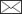# Articles containing the keyword 'two-phase sampling'.

## Category: Research article

article id 596, category Research article
(2001). Improving multi-source forest inventory by weighting auxiliary data sources. Silva Fennica vol. 35 no. 2 article id 596. https://doi.org/10.14214/sf.596
A two-phase sampling design has been applied to forest inventory. First, a large number of first phase sample plots were defined with a square grid in a geographic coordinate system for two study areas of about 1800 and 4500 ha. The first phase sample plots were supplied by auxiliary data of Landsat TM and IRS-1C with principal component transformation for stratification and drawing the second phase sample (field sample). Proportional allocation was used to draw the second phase sample. The number of field sample plots in the two study areas was 300 and 380. The local estimates of five continuous forest stand variables, mean diameter, mean height, age, basal area, and stem volume, were calculated for each of the first phase sample plots. This was done separately by using one auxiliary data source at a time together with the field sample information. However, if the first phase sample plot for which the stand variables were to be estimated was also a field sample plot, the information of that field sample plot was eliminated according to the cross validation principle. This was because it was then possible to calculate mean square errors of estimates related to a specific auxiliary data source. The procedure produced as many estimates for each first phase sample plot and forest stand variable as was the number of auxiliary data sources, i.e. seven estimates: These were based on Landsat TM, IRS-1C, digitized aerial photos, ocular stereoscopic interpretation from aerial photographs, data from old forest inventory made by compartments, Landsat TM95–TM89 difference image and IRS96–TM95 difference image. The final estimates were calculated as weighted averages where the weights were inversely proportional to mean square errors. The alternative estimates were calculated by applying simple rules based on knowledge and the outliers were defined. The study shows that this kind of system for finding outliers for elimination and a weighting procedure improves the accuracy of stand variable estimation.
• Tuominen, Finnish Forest Research Institute, Unioninkatu 40 A, FIN-00170 Helsinki, Finland ORCID ID:E-mail: sakari.tuominen@metla.fi• Poso, Department of Forest Resource Management, P.O. Box 27, FIN-00014 University of Helsinki, Finland ORCID ID:E-mail:
article id 669, category Research article
(1999). Weighting alternative estimates when using multi-source auxiliary data for forest inventory. Silva Fennica vol. 33 no. 1 article id 669. https://doi.org/10.14214/sf.669
Five auxiliary data sources (Landsat TM, IRS-IC, digitized aerial photographs, visual photo-interpretation and old forest compartment information) applying three study areas and three estimators, two-phase sampling with stratification, the k nearest neighbors and regression estimator, were examined. Auxiliary data were given for a high number of sample plots, which are here called first phase sample plots. The plots were distributed using a systematic grid over the study areas. Some of the plots were then measured in the field for the necessary ground truth. Each auxiliary data source in combination with field sample information was applied to produce a specific estimator for five forest stand characteristics: mean diameter, mean height, age, basal area, and volume of the growing stock. When five auxiliary data sources were used, each stand characteristic and each first phase sample plot were supplied with five alternative estimates with three alternative estimators. Mean square errors were then calculated for each alternative estimator using the cross validation method. The final estimates were produced by weighting alternative estimates inversely according to the mean square errors related to the corresponding estimator. The result was better than the final estimate of any of the single estimators. The improvement over the best single estimate, as measured in mean square error, was 16.9% on average for all five forest stand characteristics. The improvement was fairly equal for all five forest stand characteristics. Only minor differences among the accuracies of the three alternative estimators were recorded.
• Poso, Department of Forest Resource Management, P.O. Box 24 (Unioninkatu 40 B), FIN-00014 University of Helsinki, Finland ORCID ID:E-mail: simo.poso@helsinki.fi• Wang, Department of Forest Resource Management, P.O. Box 24 (Unioninkatu 40 B), FIN-00014 University of Helsinki, Finland ORCID ID:E-mail:
• Tuominen, Department of Forest Resource Management, P.O. Box 24 (Unioninkatu 40 B), FIN-00014 University of Helsinki, Finland ORCID ID:E-mail:

Register
Click this link to register for Silva Fennica submission and tracking system.
Log in
If you are a registered user, log in to save your selected articles for later access.
Contents alert
Sign up to receive alerts of new content
Your selected articles